Technical Article

# Visualizing Transformer Behavior: Drawing Phasor Diagrams and Equivalent Circuits

July 09, 2023 by Amna Ahmad

Phasor diagrams are graphical representations that help analyze the behavior of electrical quantities in AC circuits. When applied to transformers, phasor diagrams demonstrate the relationship between current, voltage, and power in both load and no-load conditions.##### Image used courtesy of Adobe Stock

Equivalent circuits can be used to represent transformers in a simplified manner. The equivalent circuit for a no-load condition includes the magnetizing branch, which accounts for the magnetizing current and core losses. In the load condition, the equivalent circuit consists of the series impedance branch (including resistance and reactance), representing the voltage drop across the transformer windings (primary and secondary) and the load impedance.

When the output terminals of a transformer are not connected, it is known as a no-load condition. During this state, no current flows through the secondary windings. However, an alternating voltage applied to the input terminals induces a small primary current, which generates the magnetic flux in the core. This current is commonly referred to as the magnetizing current. Hysteresis loss occurs when the magnetic flux in a core continuously increases to its peak level in one direction, reverses to a peak in the opposite direction, and the core absorbs energy. Eddy currents are also induced in the transformer core due to the alternating magnetic flux, which results in additional energy dissipation in the core.

To minimize hysteresis loss, it is crucial to use magnetic material with a narrow hysteresis loop, and the core of the transformer is built of laminations to minimize eddy current loss. However, the laminated construction introduces air gaps in the core, as shown in Figure 1, and the magnetizing current must create a flux in both the core and the air gaps. In addition to these losses, there is also an I2R loss in the transformer primary windings that must be supplied. As a result, the primary no-load current comprises the magnetizing current and the current required to provide core losses.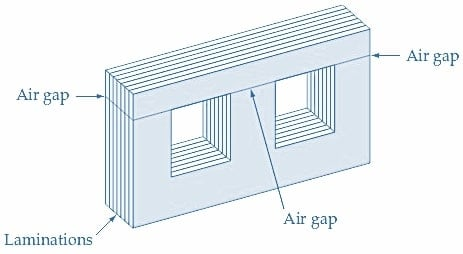##### Figure 1. Energy losses occur in a transformer core because of eddy currents and air gaps. The primary no-load current must supply these core losses as well as create a magnetic flux in the core. Image used courtesy of Amna Ahmad

The transformer's circuit and an approximate phasor diagram under no-load conditions are illustrated in Figure 2. Since the transformer windings are inductive, the input voltage VP leads the magnetizing current (Imag) by 90°.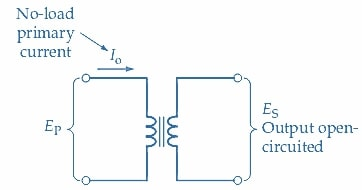##### (a) Circuit of a transformer with output open-circuited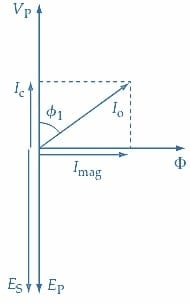##### Figure 2. Circuit and phasor diagram for a transformer on no-load. The core flux (ɸ) is in phase with the magnetizing current (Imag). The windings are inductive, so Imag lags the primary voltage (VP) by 90°. Image used courtesy of Amna Ahmad

The magnetizing current causes the flux (ɸ) to fluctuate, resulting in the flux phasor being in phase with Imag. IC is the portion of the primary current responsible for providing core losses and a minor power loss in the primary winding. The total power loss is equal to (IC×VP), and as a result, IC is in phase with VP. The no-load primary current (Io) is the result of the phasor sum of Imag and IC. Given that IC is typically much smaller than Imag, the no-load power factor (cos ɸ1) is quite small. The voltages induced in the secondary and primary windings, ES and EP, respectively, lag the flux by 90°, and thus the ES and EP phasors are drawn opposite to the VP phasor. In Figure 2, ES and EP are shown as equal voltages (i.e., assuming a 1:1 transformer). An approximation in the phasor diagram of Figure 2 occurs because EP has been taken as exactly equal and opposite to VP. In fact, EP is equal and opposite to the phasor sum of VP, and the winding voltage drops due to lo. This becomes more evident when a transformer on-load is considered.

Figure 3 shows the equivalent circuit for the transformer when it is under no-load condition. In this circuit, an ideal transformer is used to replace the actual transformer, and it has a parallel resistance Ro and inductive reactance Xo on its primary side. Ro is used to represent core losses, and the current IC that supplies the core losses flows through it. Xo represents a loss-free coil that carries the magnetizing current Imag. Hence, Ra, Xo, and the ideal transformer together simulate the actual transformer's no-load state.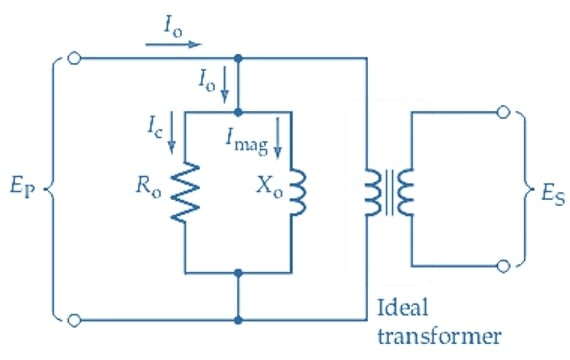##### Figure 3. The no-load equivalent circuit of a transformer is made up of an ideal (no loss) transformer with its primary in parallel with a resistance (Ro) representing core losses and an inductive reactance (Xo) to represent the winding inductance. Image used courtesy of Amna Ahmad

When a load is connected to the secondary winding of a transformer, a current flows through the secondary circuit. As illustrated in Figure 4, the secondary current tends to generate a flux ɸ2 in the transformer core. To supply the secondary current, the current must flow in the primary winding. The primary current generates a flux ɸ1 which is exactly equal and opposite to ɸ2. Thus, ɸ1 and ɸ2 cancel each other out, and the core flux remains at the level set up by the magnetizing current.

In Figure 4, it is illustrated that when currents pass through the primary and secondary windings of a transformer, not all of the flux created by the currents flows through the iron core. Some of the flux passes through the air surrounding each coil, which is known as leakage flux. Since the magnetic path through the iron core has a much lower reluctance than the air path around each coil, the amount of leakage flux is usually small. However, the leakage flux still links with the winding turns in each coil, resulting in the generation of EMFs that oppose the current flow through each coil. This is similar to an unwanted inductance connected in series with each winding, which is referred to as leakage inductance.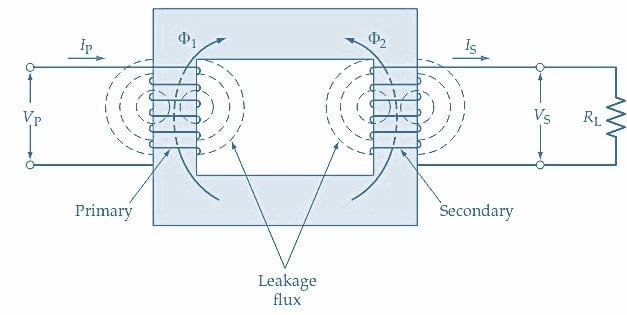### Complete Equivalent Circuit

Figure 5 shows the complete equivalent circuit of a transformer. It includes the leakage inductances of the primary and secondary windings represented by inductive reactance XP and XS, respectively, as well as the winding resistances RP and RS. RS and XS are connected in series with the secondary winding, causing a voltage drop when a load current is present. Similarly, RP and XP are connected in series with the primary winding, resulting in voltage drops when a primary current flows. Ro and Xo remain in parallel with the primary to mimic the no-load losses and magnetizing current, respectively.### Phasor Diagram for Secondary Circuit

The phasor diagram for the secondary circuit of a transformer under load is shown in Figure 6. Referring to Figures 5 and 6(a), Vo is the voltage at the transformer output terminals, and IS is the secondary (load) current. For a load with a lagging phase angle (ɸO), the IS phasor is shown lagging the Vo phasor by angle ɸo. The secondary current flows through RS and XS and produces voltage drops: ISRS and ISXS. The resistive voltage drop (ISRS) is in phase with IS, and the inductive voltage drop (ISXS) leads the current by 90°, as shown in Figure 6(a).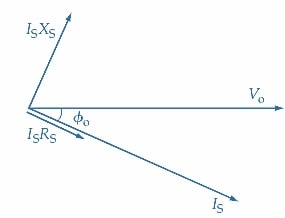##### (a) Partial phasor diagram for a secondary circuit with an inductive (lagging ɸ) load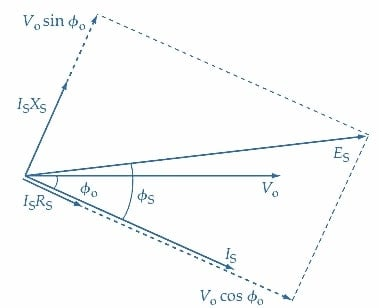##### Figure 6. Phasor diagram for the secondary of a transformer under load. Secondary current IS lags the output voltage Vo by the load phase angle ɸo. (ISXS) and (ISRS) are the voltage drops across XS and RS. The secondary induced voltage ES is the phasor sum of Vo, (ISXS), and (ISRS). Image used courtesy of Amna Ahmad

In Figure 6(b), the phasors of VO, IS, ISXS, and ISRS are reproduced from Figure 6(a). The secondary induced voltage ES (also see Figure 5) is the phasor sum of Vo, ISRS, and ISXS.

$E_{S}=\sqrt{(V_{O}\,cos\,cos\,\Phi_{O}+I_{S}R_{S})^{2}+(V_{O}\,sin\,sin\,\Phi_{O}+I_{S}X_{S})^{2}}(1)$

and the phase angle between ES and IS is

$\Phi_{S}=\Big( \frac{V_{O}sinsin\Phi_{O}+I_{S}X_{S}}{V_{O}+I_{S}X_{S}}\Big)(2)$

Since the secondary induced voltage always lags 90° behind the core flux ɸ, the core flux phasor may be drawn 90° ahead of ES.

### Phasor Diagram for the Primary

The phasor diagram for the transformer primary may be constructed in a similar way to that just discussed for the secondary. In this case, it is necessary to commence with the voltage EP and current IP right at the primary winding (see Figure 5). EP and IP' may be calculated from the knowledge of the turn’s ratio and the secondary current and voltage. The phase angle between EP and IP' is ɸS, the phase angle of the secondary circuit.

Referring to Figure 7(a), the -EP phasor is first drawn horizontally, and the IP' current phasor is drawn at an angle of ɸS lagging -EP. Note that because EP is the voltage induced in the primary winding by the changing core flux, it is equal and opposite to the component of the applied voltage at the (ideal)transformer primary winding. Consequently, the applied voltage component is -EP.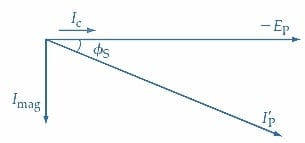##### (a) IP' lags – EP by ɸS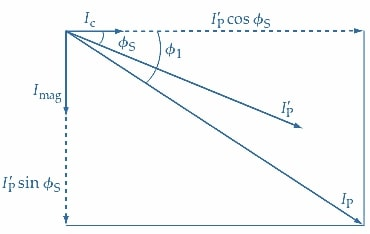##### (b) IP is the phasor sum of IP', Imag, and IC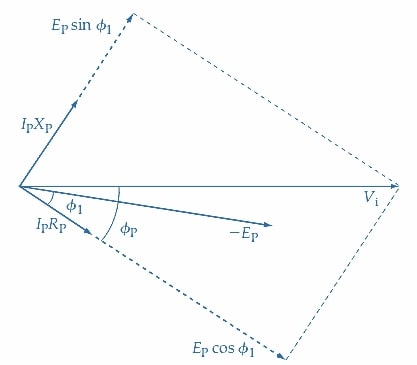##### Figure 7. Phasor diagram for the primary of a transformer under load. The primary current (IP') lags the primary voltage (EP) by the secondary phase angle (ɸS). No-load currents IC and Imag are also included, and the total primary current (IP) is the phasor sum of IP', IC, and Imag. The supply voltage (Vi) is the phasor sum of -EP, (IPXP), and (IPRP). Image used courtesy of Amna Ahmad

As well as IP', the no-load current Io (composed of IC and Imag) must be supplied. The actual current drawn from the supply is IP, which is the phasor sum of IP', IC, and Imag as shown in Figures 5 and 7(b).

$I_{P}\sqrt{(I^{'}_{P}\,cos\,cos\,\Phi_{S}+I_{C})^{2}}+(I^{'}_{P}+I_{mag})^{2}(3)$

And

$\Phi_{1}=\Big(\frac{I^{'}_{P}sin\,sin\,\Phi_{S}+I_{mag}}{I^{'}_{P}+I_{C}}\Big)(4)$

The primary current (IP) produces a voltage drop along RP and XP (Figure 5). The IPRP phasor is in phase with IP, and the IPXP phasor leads IP by 90°, as shown in Figure 7(c). The phasor sums of IPRP, IPXP, and -EP gives the supply voltage Vi. As illustrated, the primary input phase angle is ɸP, the angle between Vi and IP

Given the secondary load and the parameters of the transformer equivalent circuit, the primary input voltage and current can be calculated using the phasor diagram.

### Takeaways of Transformer Phasor Diagrams and Equivalent Circuits

The phasor diagram and equivalent circuit of a transformer provide valuable information about the electrical behavior of the transformer under both load and no-load conditions. These concepts help analyze voltage regulation, power losses, and transformer efficiency. Therefore, it is crucial to have a solid understanding of the phasor diagram and equivalent circuit to ensure the safe and efficient operation of transformers.